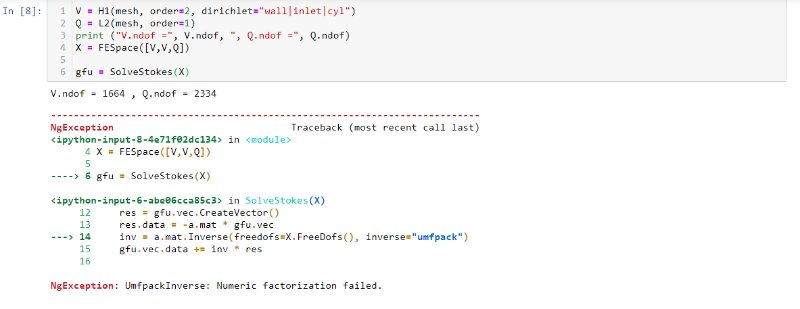# Different results when running Stokes equation example

I tried to run the example in 2.6 Stokes equationsThe results that I got in line 8 was different from what it was in the documentation.

## [code]V.ndof = 1664 , Q.ndof = 2334

NgException Traceback (most recent call last)
in
4 X = FESpace([V,V,Q])
5
----> 6 gfu = SolveStokes(X)

in SolveStokes(X)
12 res = gfu.vec.CreateVector()
13 res.data = -a.mat * gfu.vec
—> 14 inv = a.mat.Inverse(freedofs=X.FreeDofs(), inverse=“umfpack”)
15 gfu.vec.data += inv * res
16

NgException: UmfpackInverse: Numeric factorization failed.[/code]
It should be V.ndof = 1660 , Q.ndof = 2328.

Could you tell me why i got this error and how to fix this problem? Thank you so much.

easy fix: don’t use continuous P2 for velocity, and discontinuous P1 for pressure.

NGSolve and Umfpack are correctly telling that this is an unstable method (even leading to a singular matrix).

Joachim

I’m sorry what I meant was V.ndof = 1664 , Q.ndof = 2334 in my results, and V.ndof = 1660 , Q.ndof = 2328 in the documentation while the codes are the same.

Since the output from the docu was created, the mesh generation will have probably changed slightly, such that the slightly different numbers of dofs is be due do slightly different generated meshes.

I assume you have an older ngsolve version installed, since your output matches the one of older ngsolve documentations.

Thank you. I installed the newest version and I got the same result in the documentation.